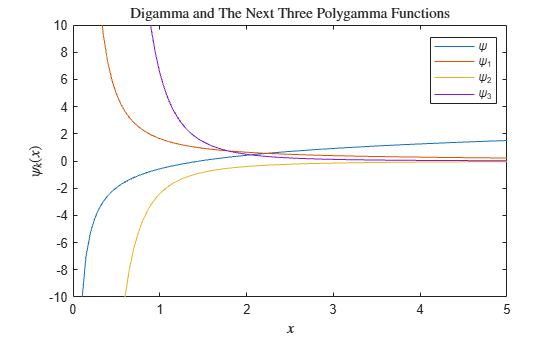Main Content

# psi

Digamma and polygamma functions

## Syntax

Y = psi(X)
Y = psi(k,X)

## Description

example

Y = psi(X) evaluates the digamma function for each element of array X, which must be real and nonnegative.

example

Y = psi(k,X) evaluates the polygamma function of X, which is the kth derivative of the digamma function at X. Thus, psi(0,X) is the digamma function, psi(1,X) is the trigamma function, psi(2,X) is the tetragamma function, and so on.

## Examples

collapse all

Use the psi function to evaluate the Euler-Mascheroni constant $\gamma$, also known as Euler's constant.

format long Y = -psi(1)
Y = 0.577215664901532 

Evaluate the trigamma function of 2.

format long Y1 = psi(1,2)
Y1 = 0.644934066848226 

Check that the result is equal to ${\pi }^{2}/6-1$.

Y2 = pi^2/6 - 1
Y2 = 0.644934066848226 
isequal(Y1,Y2)
ans = logical 1 

Define the domain.

X = 0:0.05:5;

Calculate the digamma and the next three polygamma functions.

Y = zeros(4,101); for i = 0:3 Y(i+1,:) = psi(i,X); end

Plot the digamma and the next three polygamma functions.

plot(X,Y) axis([0 5 -10 10]) legend('\psi','\psi_1','\psi_2','\psi_3','Location','Best') title('Digamma and The Next Three Polygamma Functions','interpreter','latex') xlabel('$x$','interpreter','latex') ylabel('$\psi_k(x)$','interpreter','latex')## Input Arguments

collapse all

Input, specified as a scalar, vector, matrix, or multidimensional array of nonnegative real numbers. X cannot be sparse.

Data Types: single | double

Order of derivative, specified as a nonnegative integer scalar. k must be smaller than 231-1.

Data Types: single | double

## More About

collapse all

### Digamma Function

The digamma function is the first derivative of the logarithm of the gamma function:

$\psi \left(x\right)=\frac{d}{dx}\mathrm{ln}\Gamma \left(x\right)=\frac{{\Gamma }^{\prime }\left(x\right)}{\Gamma \left(x\right)}.$

### Polygamma Function

The polygamma function of the order k is the (k + 1)th derivative of the logarithm of the gamma function:

${\psi }^{\left(k\right)}\left(x\right)=\frac{{d}^{k+1}}{d{x}^{k+1}}\mathrm{ln}\Gamma \left(x\right)=\frac{{d}^{k}}{d{x}^{k}}\psi \left(x\right).$

 Abramowitz, M. and I. A. Stegun, Handbook of Mathematical Functions, Dover Publications, 1965, Sections 6.3 and 6.4.

Download ebook